# Mean State

Period Mean (original grids) [Watt m-2]
Model Period Mean (intersection) [Watt m-2]
Model Period Mean (complement) [Watt m-2]
Benchmark Period Mean (intersection) [Watt m-2]
Benchmark Period Mean (complement) [Watt m-2]
Bias [Watt m-2]
RMSE [Watt m-2]
Phase Shift [months]
Bias Score 
RMSE Score 
Seasonal Cycle Score 
Spatial Distribution Score 
Interannual Variability Score 
Overall Score 
Benchmark [-] 179.
CLM4 [-] 182. 182. 0.00 177. 216. 5.16 16.6 0.531 0.64 0.52 0.93 0.92 0.73 0.71
CLM4.5 [-] 183. 183. 0.00 177. 216. 5.50 16.8 0.545 0.62 0.52 0.93 0.93 0.73 0.71
CLM5 [-] 183. 183. 0.00 177. 216. 5.83 16.7 0.533 0.63 0.52 0.93 0.94 0.73 0.71
Period Mean (original grids) [Watt m-2]
Model Period Mean (intersection) [Watt m-2]
Model Period Mean (complement) [Watt m-2]
Benchmark Period Mean (intersection) [Watt m-2]
Benchmark Period Mean (complement) [Watt m-2]
Bias [Watt m-2]
RMSE [Watt m-2]
Phase Shift [months]
Bias Score 
RMSE Score 
Seasonal Cycle Score 
Spatial Distribution Score 
Interannual Variability Score 
Overall Score 
Benchmark [-] 189.
CLM4 [-] 193. 193. 0.00 188. 224. 5.16 15.8 0.379 0.70 0.54 0.97 1.0 0.79 0.75
CLM4.5 [-] 194. 194. 0.00 188. 224. 6.02 16.3 0.394 0.68 0.53 0.97 1.0 0.79 0.75
CLM5 [-] 195. 195. 0.00 188. 224. 6.89 16.6 0.458 0.66 0.53 0.95 1.0 0.78 0.74
Period Mean (original grids) [Watt m-2]
Model Period Mean (intersection) [Watt m-2]
Model Period Mean (complement) [Watt m-2]
Benchmark Period Mean (intersection) [Watt m-2]
Benchmark Period Mean (complement) [Watt m-2]
Bias [Watt m-2]
RMSE [Watt m-2]
Phase Shift [months]
Bias Score 
RMSE Score 
Seasonal Cycle Score 
Spatial Distribution Score 
Interannual Variability Score 
Overall Score 
Benchmark [-] 194.
CLM4 [-] 196. 196. 0.00 192. 221. 3.08 18.5 0.555 0.73 0.56 0.92 0.96 0.67 0.73
CLM4.5 [-] 196. 196. 0.00 192. 221. 3.59 18.8 0.575 0.72 0.56 0.92 0.96 0.67 0.73
CLM5 [-] 197. 197. 0.00 192. 221. 3.85 18.7 0.529 0.72 0.56 0.93 0.96 0.67 0.73
Period Mean (original grids) [Watt m-2]
Model Period Mean (intersection) [Watt m-2]
Model Period Mean (complement) [Watt m-2]
Benchmark Period Mean (intersection) [Watt m-2]
Benchmark Period Mean (complement) [Watt m-2]
Bias [Watt m-2]
RMSE [Watt m-2]
Phase Shift [months]
Bias Score 
RMSE Score 
Seasonal Cycle Score 
Spatial Distribution Score 
Interannual Variability Score 
Overall Score 
Benchmark [-] 81.3
CLM4 [-] 66.3 66.4 0.00 80.9 86.2 -14.7 25.3 0.338 0.81 0.72 0.98 0.94 0.77 0.82
CLM4.5 [-] 71.3 71.4 0.00 80.9 86.2 -10.5 21.0 0.213 0.85 0.75 0.99 0.89 0.71 0.82
CLM5 [-] 72.8 72.9 0.00 80.9 86.2 -9.00 20.1 0.218 0.87 0.75 0.99 0.92 0.70 0.83
Period Mean (original grids) [Watt m-2]
Model Period Mean (intersection) [Watt m-2]
Model Period Mean (complement) [Watt m-2]
Benchmark Period Mean (intersection) [Watt m-2]
Benchmark Period Mean (complement) [Watt m-2]
Bias [Watt m-2]
RMSE [Watt m-2]
Phase Shift [months]
Bias Score 
RMSE Score 
Seasonal Cycle Score 
Spatial Distribution Score 
Interannual Variability Score 
Overall Score 
Benchmark [-] 189.
CLM4 [-] 177. 177. 0.00 183. 210. -1.97 20.6 0.688 0.61 0.45 0.91 0.72 0.71 0.64
CLM4.5 [-] 178. 177. 0.00 183. 210. -1.82 20.6 0.686 0.61 0.45 0.91 0.71 0.71 0.64
CLM5 [-] 177. 177. 0.00 183. 210. -2.07 20.5 0.665 0.61 0.46 0.91 0.73 0.71 0.65
Period Mean (original grids) [Watt m-2]
Model Period Mean (intersection) [Watt m-2]
Model Period Mean (complement) [Watt m-2]
Benchmark Period Mean (intersection) [Watt m-2]
Benchmark Period Mean (complement) [Watt m-2]
Bias [Watt m-2]
RMSE [Watt m-2]
Phase Shift [months]
Bias Score 
RMSE Score 
Seasonal Cycle Score 
Spatial Distribution Score 
Interannual Variability Score 
Overall Score 
Benchmark [-] 105.
CLM4 [-] 102. 102. 0.00 104. 127. -2.21 15.7 0.0825 0.94 0.81 0.99 0.98 0.65 0.86
CLM4.5 [-] 99.6 99.6 0.00 104. 127. -4.37 17.6 0.0854 0.92 0.79 0.99 0.98 0.65 0.86
CLM5 [-] 100. 100. 0.00 104. 127. -3.81 16.7 0.0856 0.93 0.80 0.99 1.0 0.64 0.86
Period Mean (original grids) [Watt m-2]
Model Period Mean (intersection) [Watt m-2]
Model Period Mean (complement) [Watt m-2]
Benchmark Period Mean (intersection) [Watt m-2]
Benchmark Period Mean (complement) [Watt m-2]
Bias [Watt m-2]
RMSE [Watt m-2]
Phase Shift [months]
Bias Score 
RMSE Score 
Seasonal Cycle Score 
Spatial Distribution Score 
Interannual Variability Score 
Overall Score 
Benchmark [-] 140.
CLM4 [-] 135. 134. 0.00 139. 154. -4.57 17.4 0.219 0.88 0.74 0.98 0.99 0.62 0.83
CLM4.5 [-] 134. 134. 0.00 139. 154. -5.35 17.6 0.209 0.87 0.74 0.98 0.99 0.62 0.83
CLM5 [-] 136. 135. 0.00 139. 154. -3.72 16.9 0.242 0.89 0.74 0.98 1.0 0.62 0.83
Period Mean (original grids) [Watt m-2]
Model Period Mean (intersection) [Watt m-2]
Model Period Mean (complement) [Watt m-2]
Benchmark Period Mean (intersection) [Watt m-2]
Benchmark Period Mean (complement) [Watt m-2]
Bias [Watt m-2]
RMSE [Watt m-2]
Phase Shift [months]
Bias Score 
RMSE Score 
Seasonal Cycle Score 
Spatial Distribution Score 
Interannual Variability Score 
Overall Score 
Benchmark [-] 180.
CLM4 [-] 174. 174. 0.00 178. 210. -2.65 19.1 0.305 0.80 0.62 0.98 0.96 0.64 0.77
CLM4.5 [-] 174. 174. 0.00 178. 210. -2.23 19.1 0.279 0.80 0.62 0.98 0.95 0.64 0.77
CLM5 [-] 175. 175. 0.00 178. 210. -1.80 18.7 0.253 0.81 0.62 0.98 0.92 0.63 0.76
Period Mean (original grids) [Watt m-2]
Model Period Mean (intersection) [Watt m-2]
Model Period Mean (complement) [Watt m-2]
Benchmark Period Mean (intersection) [Watt m-2]
Benchmark Period Mean (complement) [Watt m-2]
Bias [Watt m-2]
RMSE [Watt m-2]
Phase Shift [months]
Bias Score 
RMSE Score 
Seasonal Cycle Score 
Spatial Distribution Score 
Interannual Variability Score 
Overall Score 
Benchmark [-] 74.6
CLM4 [-] 58.5 58.9 0.00 74.6 74.8 -16.2 22.4 0.224 0.78 0.72 0.99 0.93 0.72 0.81
CLM4.5 [-] 61.2 61.6 0.00 74.6 74.8 -13.8 20.5 0.265 0.81 0.73 0.98 0.92 0.68 0.81
CLM5 [-] 64.0 64.4 0.00 74.6 74.8 -11.2 19.0 0.311 0.84 0.74 0.98 0.93 0.67 0.82
Period Mean (original grids) [Watt m-2]
Model Period Mean (intersection) [Watt m-2]
Model Period Mean (complement) [Watt m-2]
Benchmark Period Mean (intersection) [Watt m-2]
Benchmark Period Mean (complement) [Watt m-2]
Bias [Watt m-2]
RMSE [Watt m-2]
Phase Shift [months]
Bias Score 
RMSE Score 
Seasonal Cycle Score 
Spatial Distribution Score 
Interannual Variability Score 
Overall Score 
Benchmark [-] 193.
CLM4 [-] 191. 191. 0.00 193. 202. -3.08 17.9 0.169 0.86 0.71 0.99 1.0 0.61 0.81
CLM4.5 [-] 191. 191. 0.00 193. 202. -3.11 17.9 0.154 0.86 0.71 0.99 1.0 0.62 0.81
CLM5 [-] 192. 192. 0.00 193. 202. -1.99 18.1 0.222 0.85 0.70 0.98 1.0 0.61 0.81
Period Mean (original grids) [Watt m-2]
Model Period Mean (intersection) [Watt m-2]
Model Period Mean (complement) [Watt m-2]
Benchmark Period Mean (intersection) [Watt m-2]
Benchmark Period Mean (complement) [Watt m-2]
Bias [Watt m-2]
RMSE [Watt m-2]
Phase Shift [months]
Bias Score 
RMSE Score 
Seasonal Cycle Score 
Spatial Distribution Score 
Interannual Variability Score 
Overall Score 
Benchmark [-] 120.
CLM4 [-] 112. 112. 0.00 117. 151. -2.82 17.7 0.335 0.89 0.79 0.98 0.99 0.64 0.85
CLM4.5 [-] 113. 113. 0.00 117. 151. -1.86 16.6 0.303 0.91 0.80 0.98 1.0 0.63 0.85
CLM5 [-] 113. 113. 0.00 117. 151. -1.95 16.4 0.358 0.91 0.80 0.98 1.0 0.62 0.85
Period Mean (original grids) [Watt m-2]
Model Period Mean (intersection) [Watt m-2]
Model Period Mean (complement) [Watt m-2]
Benchmark Period Mean (intersection) [Watt m-2]
Benchmark Period Mean (complement) [Watt m-2]
Bias [Watt m-2]
RMSE [Watt m-2]
Phase Shift [months]
Bias Score 
RMSE Score 
Seasonal Cycle Score 
Spatial Distribution Score 
Interannual Variability Score 
Overall Score 
Benchmark [-] 168.
CLM4 [-] 135. 135. 0.00 143. 180. -6.91 19.6 0.271 0.78 0.67 0.97 0.96 0.63 0.78
CLM4.5 [-] 136. 136. 0.00 143. 180. -6.33 19.0 0.272 0.78 0.67 0.97 0.97 0.63 0.78
CLM5 [-] 137. 137. 0.00 143. 180. -5.85 18.9 0.278 0.79 0.67 0.97 0.97 0.62 0.78
Period Mean (original grids) [Watt m-2]
Model Period Mean (intersection) [Watt m-2]
Model Period Mean (complement) [Watt m-2]
Benchmark Period Mean (intersection) [Watt m-2]
Benchmark Period Mean (complement) [Watt m-2]
Bias [Watt m-2]
RMSE [Watt m-2]
Phase Shift [months]
Bias Score 
RMSE Score 
Seasonal Cycle Score 
Spatial Distribution Score 
Interannual Variability Score 
Overall Score 
Benchmark [-] 175.
CLM4 [-] 160. 160. 0.00 173. 224. -12.1 20.3 0.278 0.70 0.73 0.98 0.99 0.57 0.78
CLM4.5 [-] 161. 161. 0.00 173. 224. -11.6 20.0 0.273 0.71 0.73 0.98 0.99 0.57 0.79
CLM5 [-] 162. 162. 0.00 173. 224. -10.2 19.7 0.305 0.72 0.73 0.97 0.99 0.58 0.79
Period Mean (original grids) [Watt m-2]
Model Period Mean (intersection) [Watt m-2]
Model Period Mean (complement) [Watt m-2]
Benchmark Period Mean (intersection) [Watt m-2]
Benchmark Period Mean (complement) [Watt m-2]
Bias [Watt m-2]
RMSE [Watt m-2]
Phase Shift [months]
Bias Score 
RMSE Score 
Seasonal Cycle Score 
Spatial Distribution Score 
Interannual Variability Score 
Overall Score 
Benchmark [-] 80.0
CLM4 [-] 67.4 67.3 0.00 79.8 82.9 -13.6 22.1 0.118 0.81 0.75 0.99 0.90 0.77 0.83
CLM4.5 [-] 70.5 70.3 0.00 79.8 82.9 -11.0 19.4 0.109 0.84 0.76 0.99 0.90 0.73 0.83
CLM5 [-] 70.5 70.4 0.00 79.8 82.9 -10.9 19.6 0.0911 0.84 0.76 0.99 0.89 0.72 0.83
Period Mean (original grids) [Watt m-2]
Model Period Mean (intersection) [Watt m-2]
Model Period Mean (complement) [Watt m-2]
Benchmark Period Mean (intersection) [Watt m-2]
Benchmark Period Mean (complement) [Watt m-2]
Bias [Watt m-2]
RMSE [Watt m-2]
Phase Shift [months]
Bias Score 
RMSE Score 
Seasonal Cycle Score 
Spatial Distribution Score 
Interannual Variability Score 
Overall Score 
Benchmark [-] 205.
CLM4 [-] 192. 192. 0.00 200. 227. -6.15 20.3 0.271 0.76 0.64 0.97 0.90 0.62 0.75
CLM4.5 [-] 191. 192. 0.00 200. 227. -6.61 20.3 0.298 0.77 0.64 0.97 0.90 0.63 0.76
CLM5 [-] 192. 192. 0.00 200. 227. -6.23 20.2 0.250 0.77 0.64 0.97 0.89 0.63 0.76
Period Mean (original grids) [Watt m-2]
Model Period Mean (intersection) [Watt m-2]
Model Period Mean (complement) [Watt m-2]
Benchmark Period Mean (intersection) [Watt m-2]
Benchmark Period Mean (complement) [Watt m-2]
Bias [Watt m-2]
RMSE [Watt m-2]
Phase Shift [months]
Bias Score 
RMSE Score 
Seasonal Cycle Score 
Spatial Distribution Score 
Interannual Variability Score 
Overall Score 
Benchmark [-] 153.
CLM4 [-] 146. 146. 0.00 151. 194. -3.56 15.8 0.146 0.93 0.77 0.99 1.0 0.62 0.85
CLM4.5 [-] 145. 145. 0.00 151. 194. -4.37 15.8 0.143 0.92 0.77 0.99 1.0 0.62 0.84
CLM5 [-] 144. 144. 0.00 151. 194. -5.31 16.1 0.154 0.91 0.77 0.99 1.0 0.62 0.84
Period Mean (original grids) [Watt m-2]
Model Period Mean (intersection) [Watt m-2]
Model Period Mean (complement) [Watt m-2]
Benchmark Period Mean (intersection) [Watt m-2]
Benchmark Period Mean (complement) [Watt m-2]
Bias [Watt m-2]
RMSE [Watt m-2]
Phase Shift [months]
Bias Score 
RMSE Score 
Seasonal Cycle Score 
Spatial Distribution Score 
Interannual Variability Score 
Overall Score 
Benchmark [-] 115.
CLM4 [-] 111. 111. 0.00 114. 123. -3.01 15.2 0.0925 0.92 0.82 0.99 0.98 0.65 0.86
CLM4.5 [-] 112. 112. 0.00 114. 123. -2.43 14.6 0.0955 0.92 0.83 0.99 1.0 0.63 0.87
CLM5 [-] 111. 112. 0.00 114. 123. -2.98 14.7 0.0893 0.92 0.83 0.99 1.0 0.64 0.87
Period Mean (original grids) [Watt m-2]
Model Period Mean (intersection) [Watt m-2]
Model Period Mean (complement) [Watt m-2]
Benchmark Period Mean (intersection) [Watt m-2]
Benchmark Period Mean (complement) [Watt m-2]
Bias [Watt m-2]
RMSE [Watt m-2]
Phase Shift [months]
Bias Score 
RMSE Score 
Seasonal Cycle Score 
Spatial Distribution Score 
Interannual Variability Score 
Overall Score 
Benchmark [-] 162.
CLM4 [-] 157. 157. 0.00 162. 171. -4.35 15.9 0.143 0.92 0.81 0.99 1.0 0.65 0.86
CLM4.5 [-] 156. 156. 0.00 162. 171. -5.47 15.9 0.139 0.90 0.81 0.99 1.0 0.66 0.86
CLM5 [-] 159. 159. 0.00 162. 171. -2.78 15.1 0.159 0.93 0.81 0.99 1.0 0.65 0.86
Period Mean (original grids) [Watt m-2]
Model Period Mean (intersection) [Watt m-2]
Model Period Mean (complement) [Watt m-2]
Benchmark Period Mean (intersection) [Watt m-2]
Benchmark Period Mean (complement) [Watt m-2]
Bias [Watt m-2]
RMSE [Watt m-2]
Phase Shift [months]
Bias Score 
RMSE Score 
Seasonal Cycle Score 
Spatial Distribution Score 
Interannual Variability Score 
Overall Score 
Benchmark [-] 176.
CLM4 [-] 174. 174. 0.00 174. 226. 0.0995 17.1 0.485 0.83 0.47 0.94 0.95 0.65 0.72
CLM4.5 [-] 174. 174. 0.00 174. 226. 0.344 17.2 0.512 0.83 0.47 0.93 0.94 0.65 0.71
CLM5 [-] 174. 174. 0.00 174. 226. 0.173 17.1 0.479 0.83 0.47 0.94 0.94 0.64 0.72
Period Mean (original grids) [Watt m-2]
Model Period Mean (intersection) [Watt m-2]
Model Period Mean (complement) [Watt m-2]
Benchmark Period Mean (intersection) [Watt m-2]
Benchmark Period Mean (complement) [Watt m-2]
Bias [Watt m-2]
RMSE [Watt m-2]
Phase Shift [months]
Bias Score 
RMSE Score 
Seasonal Cycle Score 
Spatial Distribution Score 
Interannual Variability Score 
Overall Score 
Benchmark [-] 182.
CLM4 [-] 181. 181. 0.00 181. 201. -0.244 19.2 0.361 0.88 0.57 0.96 1.0 0.62 0.77
CLM4.5 [-] 181. 181. 0.00 181. 201. -0.363 19.2 0.395 0.89 0.57 0.95 1.0 0.62 0.76
CLM5 [-] 180. 181. 0.00 181. 201. -0.876 19.1 0.319 0.89 0.57 0.96 1.0 0.61 0.77
Period Mean (original grids) [Watt m-2]
Model Period Mean (intersection) [Watt m-2]
Model Period Mean (complement) [Watt m-2]
Benchmark Period Mean (intersection) [Watt m-2]
Benchmark Period Mean (complement) [Watt m-2]
Bias [Watt m-2]
RMSE [Watt m-2]
Phase Shift [months]
Bias Score 
RMSE Score 
Seasonal Cycle Score 
Spatial Distribution Score 
Interannual Variability Score 
Overall Score 
Benchmark [-] 176.
CLM4 [-] 174. 173. 0.00 176. 178. -5.68 21.2 0.306 0.84 0.70 0.97 1.0 0.61 0.80
CLM4.5 [-] 175. 174. 0.00 176. 178. -5.09 20.9 0.287 0.84 0.70 0.97 1.0 0.60 0.80
CLM5 [-] 176. 175. 0.00 176. 178. -3.76 20.8 0.301 0.84 0.70 0.97 1.0 0.60 0.80

# Temporally integrated period mean

BENCHMARK MEAN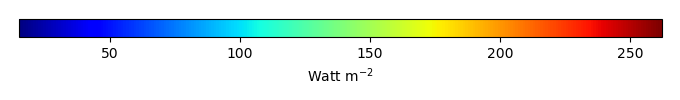MODEL MEANBIAS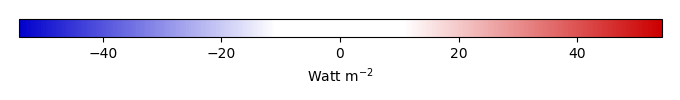BIAS SCORERMSE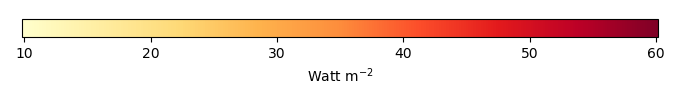RMSE SCOREBENCHMARK INTERANNUAL VARIABILITY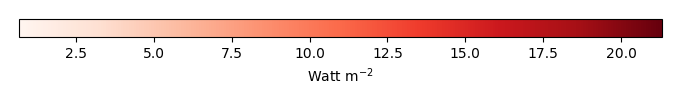MODEL INTERANNUAL VARIABILITYINTERANNUAL VARIABILITY SCOREBENCHMARK MAX MONTHMODEL MAX MONTHDIFFERENCE IN MAX MONTH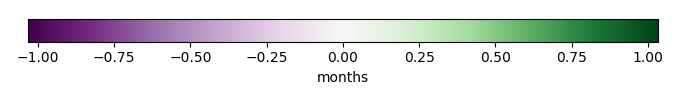SEASONAL CYCLE SCORESPATIAL TAYLOR DIAGRAMMODEL COLORS# Spatially integrated regional mean

MODEL COLORSREGIONAL MEANANNUAL CYCLEMONTHLY ANOMALYANNUAL CYCLE# All Models

BenchmarkCLM4CLM4.5CLM5# Data Information

creation_date: Tue Jul 1 08:29:23 PDT 2014

source_file: This product is generated from monthly 1 degree GEWEX SRB Radiation observations